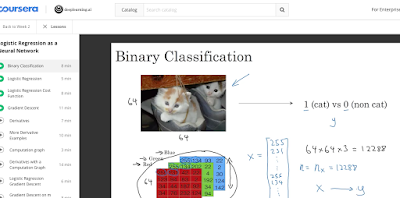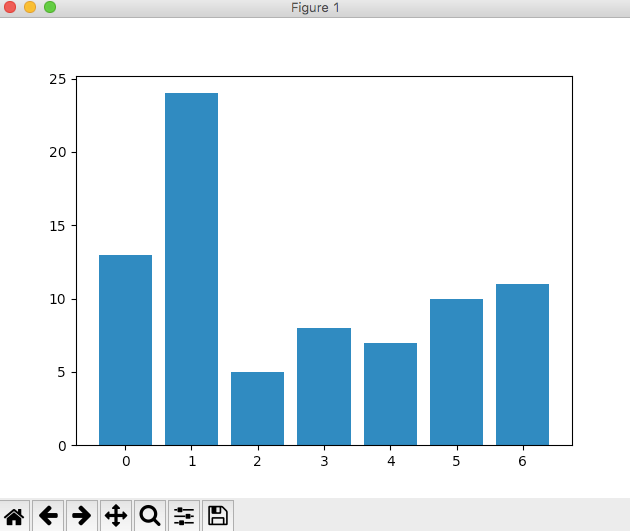## Sunday, November 12, 2017

### Matrix Vector Representation of an Image - Image Classification for Machine Learning

Each pixel intensity can be represented with RGB values - red green blue. See this Coursera Deep Learning MOOC by Andrew Ng. The RGB data of an image is known as the three 3 channels. Each is a matrix. We vectorize the RGB data into feature matrix X. Dimension length of X is = width_pixel  multiply by height_pixel multiply by number of channels 3. E.g. the digits in LSMNT  are 28 by 28 by 1 because they are black and white, so instead of 3 channels, it only has one channel.Coursera Deep Learning MOOC by Andrew Ng Convolutional Neural Net